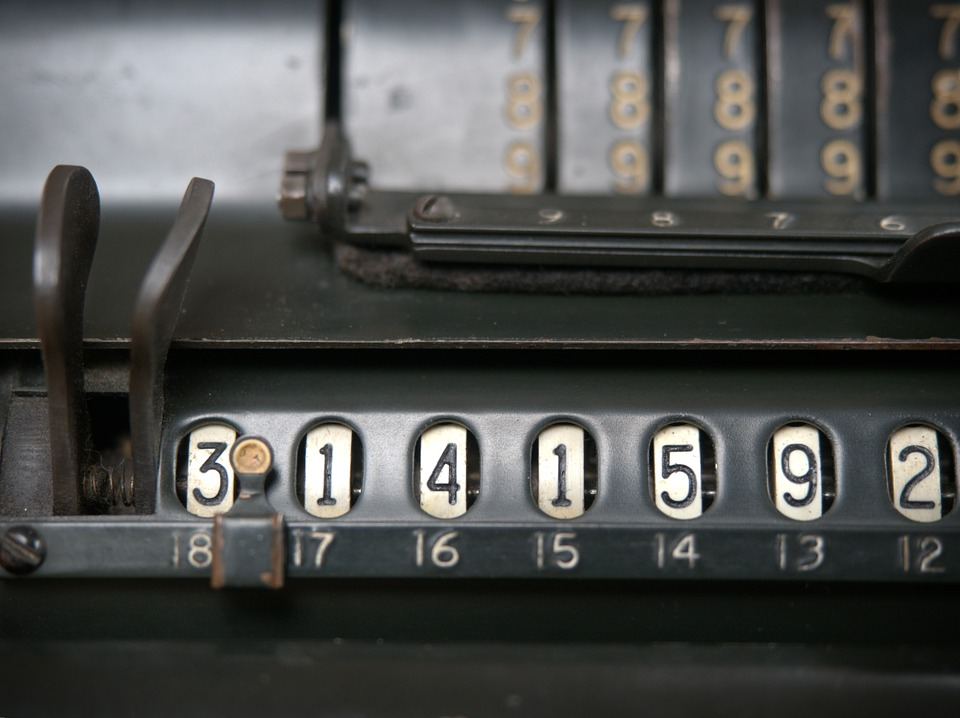• Subscribe to the blog
You must click the link in the email to verify your request.
• Explore## “Divide” and Conquer French Math VocabularyPosted by Josh Dougherty on Oct 13, 2016 in Vocabulary

You may have heard the saying that there are only 2 universal languages: music and math. While this is very debatable (love, anyone?), I thought it touched on something that we on the Transparent French blogs haven’t. We’ve talked plenty about the benefits of music in learning a foreign language, but today I wanted to focus on math. Ne vous inquiétez pas (don’t worry) – I won’t be teaching you any algebra. Math was my least favorite subject in school, so I’m not here to discuss anything more than the vocabulary 😉

When we were young, we learned comment compter (how to count). (Need a refresher on French numbers? Check out this article.) I remember in kindergarten after mastering standard counting, we learned how to compter de 2 en 2 jusqu’à 100 (to count by twos up to 100). We also learned les formes (shapes [feminine]): un cercle (a circle), un ovale (an oval), un triangle, un carré (a square), un rectangle, un losange (a diamond), un pentagone… Some of these would show up later au lycée (in high school) when we studied la géométrie (geometry), but that would be many years later.That same year, we learned simple l’addition and la soustraction (subtraction).

5 plus 3 égale 8. There’s also 5 plus 3 font 8, and 5 plus 3, ça fait 8 (familiar).
8 moins 5 égale 3 | 8 moins 5 font 3 | 8 moins 5, ça fait 3

A few years later, we took ces compétences mathématiques (these mathematical skills) a little further and learned la multiplication and la division. In English, we say 5 multiplied by 3 or 5 times 3. In French, we say 5 multiplié par 3 or 5 fois 3. In English, we say 15 divided by 3. In French, it’s 15 divisé par 3 or 15 sur 3.

5 multiplié par 3 égale 15 | 5 fois 3 font 15 | 5 fois 3, ça fait 15
15 divisé par 5 égale 3 | 15 divisé par 5 font 3 | 15 sur 5, ça fait 3

Au collège (in middle school – not college), we started learning l’algèbre (algebra – feminine). Suddenly these math problems had letters in them! But there was more: une racine carrée (square root), puissance 5 (to the 5th power), un facteur (factor), les fractions (fraction – feminine), les pourcentages (percentages – masculine), les polynômes (polynomials – masculine)…

After, we learn la géométrie: les angles (angles – masculine), une équation du second degré (quadratic equation), les théories (theories – feminine), les formes, des segments (segment – masculine), les polygones (polygon – masculine), le sinus (sine), le cosinus (cosine)… These skills were further developed with la trigonométrie (trigonometry).

In le calcul, we learn about les fonctions (functions – feminine), les dérivées (derivatives – feminine), le théorème des accroissements finis (the Mean Value Theorem), et comment trouver la limite (and how to find the limit)…

Here’s a more complete list:

Subjects
l’algèbre – algebra
le calcul – calculus
la géométrie – geometry
la trigonométrie – trigonometry

Basic Operations
la somme – sum
la soustraction – subtraction
la différence – difference
la multiplication – multiplication
le produit – product
la division – division
le quotient – quotient

Shapes
un cercle – circle
un ovale – oval
un triangle – triangle
un carré – square
un rectangle – rectangle
un losange – diamond
un pentagone – pentagon
un décagone – decagon
un cylindre – cylinder
un cône – cone
un dodécagone – dodecagon
un heptagon – heptagon
un hexagone – hexagon
un polygone – polygon

Numbers & Lines
un nombre – number
un entier – integer
pair – even
impair – odd
un nombre négatif – negative number
un nombre imaginaire pur – imaginary number
une fraction – fraction
un pourcentage – percent
un exposant – exponent
puissance 4 – to the 4th power
un logarithme – logarithm
le cube / au cube – cube / cubed
le carré / au carré – square / squared
to square – mettre au carré
une racine cubique – cube root
une racine carrée – square root
une équation – equation
une fraction – fraction
une tagente – tangent

Functions, Measurements, & Angles
le sinus – sine
le cosinus – cosine
un angle – angle
une fonction – function
un logarithme – logarithm
un angle droit – right angle
mesurer – to measure
la measure – measurementKeep learning French with us!

Build vocabulary, practice pronunciation, and more with Transparent Language Online. Available anytime, anywhere, on any device.### About the Author: Josh Dougherty

Just your typical francophile. If you have any topics you'd like me to discuss, feel free to let me know!